# Give the minimum size of each of the following C data structures, assuming that char values...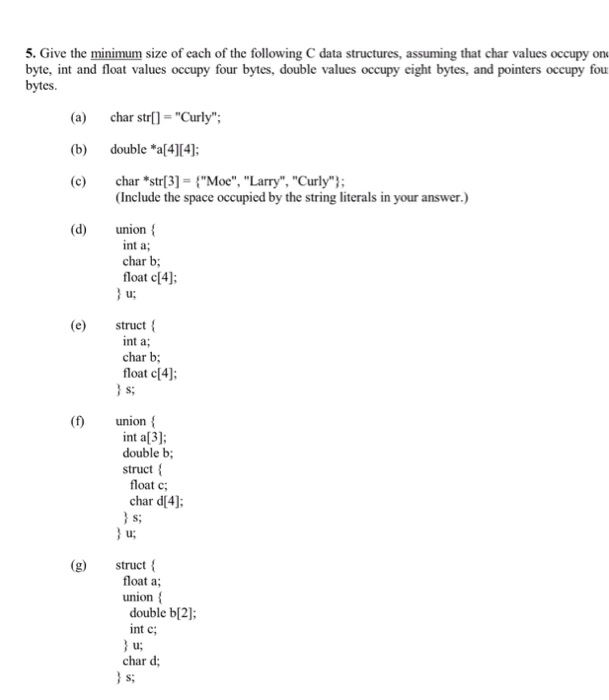Give the minimum size of each of the following C data structures, assuming that char values occupy byte, int and float values occupy four bytes, double values occupy eight bytes, and pointers occupy bytes. (a) char str[] = "Curly": (b) double *a: (c) char *str = {"Moe", "Larry", "Curly"}: (Include the space occupied by the string literals in your answer.) (d) union { int a: char b: float c: }u: (e) struct { int a: char b: float c: }s: (f) union { int a: double b: struct { float c: char d: }s: }u: (g) struct { float a: union { double b: int c: }u: char d: }s;

In c there is a method sizeof which helps you calculate the number of bytes.

eg :

#include <stdio.h>

int main()
{
char str[] = "Curly";
printf("Size of %d bytes\n",sizeof(str));

return 0;
}

Output :

6 bytes.

a) char str[] = "Curly";

Assuming char as 1 byte : size is 6 bytes

b) double *a;

size of double is 8

size of a is 16*8 = 128 bytes

c) char *str = {"Moe", "Larry", "Curly"};

char * str occupies 8 bytes.

str

8*3 = 24 bytes.

D) union {

int a;

char b;

float c;

} u;

In union, considers only the datatype with highest number of bytes : sizeof c is 4*4 = 16 bytes.

e) struct {

int a;

char b;

float c;

} s;

sum of all variables : 4+1+ (4*4) = 21 bytes.

f) union {

int a;

double b;

struct {

float c;

char d;

} s;

} u;

In the above example the total size of u is the size of u.s (which happens to be the sum of the sizes of u.s.u and u.s.d), since s is larger than both i and f. When assigning something to u.i, some parts of u.f may be preserved if u.i is smaller than u.f.

Reading from a union member is not the same as casting since the value of the member is not converted, but merely read.

Total size = 16 bytes

g) struct {

float a;

union {

double b;

int c;

} u;

char d;

} s;

Total size is 32 bytes.

#### Earn Coin

Coins can be redeemed for fabulous gifts.

Similar Homework Help Questions
• ### 2. Give the minimum size of each of the following C++ types, assuming that char values occupy one...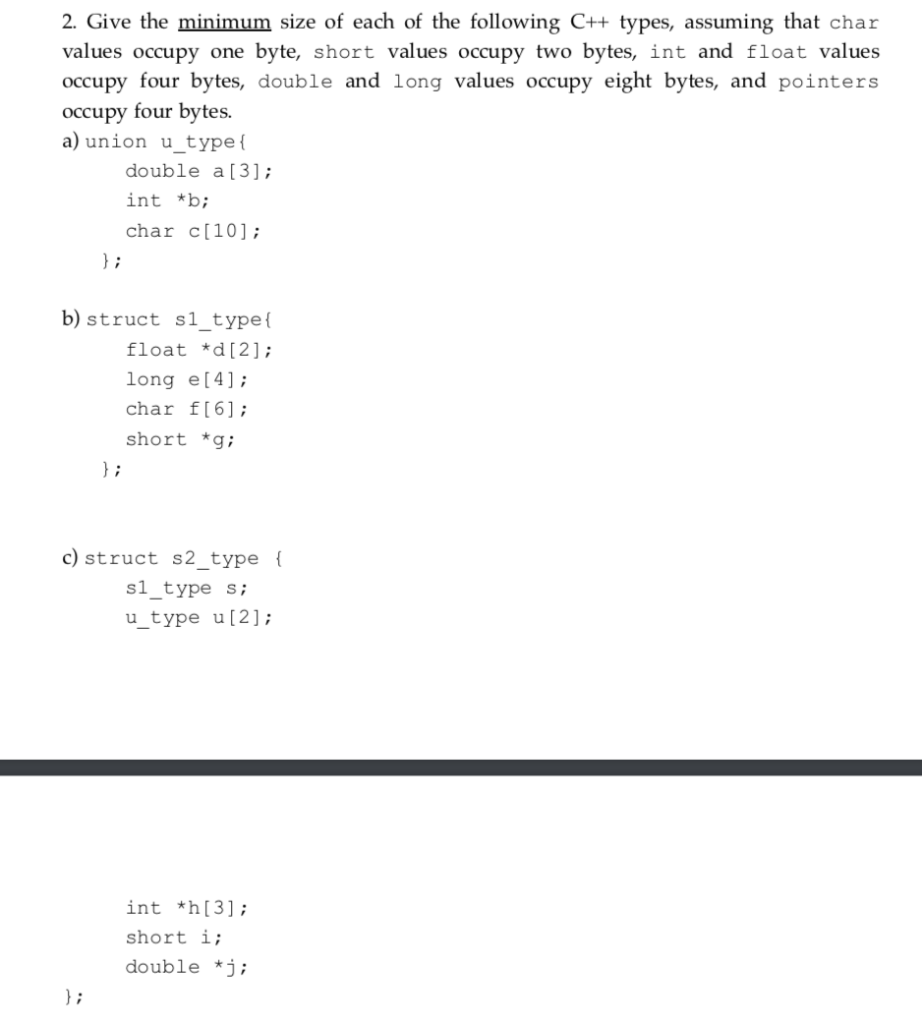2. Give the minimum size of each of the following C++ types, assuming that char values occupy one byte, short values occupy two bytes, int and float values occupy four bytes, double and long values occupy eight bytes, and pointers occupy four bytes. a) union u type ( double a; int *b; char c b) struct s1type float *d ; long e char f short *gi c) struct s2 type ( s1_type s; u type u ; int *h; short...

• ### C language not C++ 1. Write the statements to do the following: (2 pts) a. Define...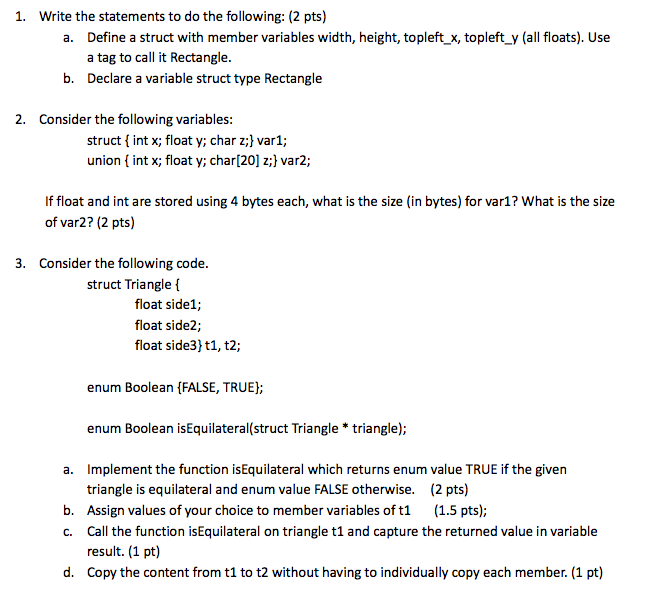C language not C++ 1. Write the statements to do the following: (2 pts) a. Define a struct with member variables width, height, topleft x, topleft y all floats). Use a tag to call it Rectangle. b. Declare a variable struct type Rectangle 2. Consider the following variables: struct int x; float y; char zi var1; union nt x; float y; char z;) var2 f float and int are stored using 4 bytes each, what is the size (in bytes)...

• ### Problem 4. (20 points) Consider the following C structs: struct S1 { char a; char b;...

Problem 4. (20 points) Consider the following C structs: struct S1 { char a; char b; char c; } struct S2 { S1 d; S1 e; S1 *f; short g; char h; double i; int j; } A. Show how the S1 struct would be in memory on a 64-bit machine. Label the bytes that belong to the various fields with their names and clearly mark the end of the struct. Use the character 'X' to indicate bytes that are...

• ### S. Modułus operator can operate upon A. double B、 float C. char D. int E. C...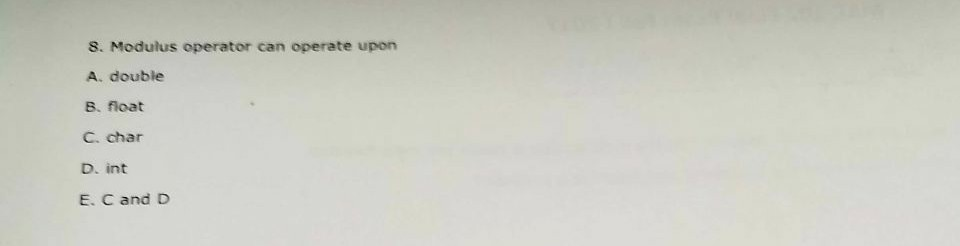S. Modułus operator can operate upon A. double B、 float C. char D. int E. C and D

• ### Provide the definition for each of the following structures and unions:

This is from chapter 10 in c how to program deitel.a) Structure inventory containing character array partName [ 30 ], integer partNumber, floating point price, integer stock and integer reorder.b) Union data containing char c, short s, long b, float f and double d.c) A structure called address that contains character arrays streetAddress[ 25 ], city[ 20 ], state[ 3 ] and zipCode[ 6 ].d) Structure student that contains arrays firstName[ 15 ] and lastName[ 15 ] variable homeAddress of...

• ### Find the error(s) in each of the following statements: This is in C

a) Assume: char str [ 5 ];Scanf( “%s”, str ); /* User types hello */b) Assume: int a[ 3 ];Printf( “\$d %d %dn”, a[ 1 ], a[ 2 ], a[ 3 ] );c) Doublef[ 3 ] = { 1, 1, 10.01, 100.001, 1000.001 };d) Assume: double d[ 2 ] [ 10 ];d[ 1, 9 ] = 2.345;

• ### QUESTION 1 In order to print the members of structures in the array, what will be...

QUESTION 1 In order to print the members of structures in the array, what will be the contents of blanks in the printf statement of the code snippet given below? #include <stdio.h> struct virus {    char signature;       char status; } v = {                               "Yankee Doodle", "Deadly",                               "Dark Avenger", "Killer"                   } ; void main( ) {       for (int i = 0; i < 2; i++)                   printf( "\n%s %s", _______________ , _____________ ); } A. signature, status B. v.signature,...

• ### State true or false: (6 x 2 = 12 pts) Using -- with a reverse iterator...

State true or false: (6 x 2 = 12 pts) Using -- with a reverse iterator moves it from the back toward the front of a list b) The body of a do-while loop is always executed at least once. c) If p points to the array element a[j], then p + i points to a[i+j]. d) Any number of pointer variables may point to the same object. In terms of both execution speed and memory usage usually iterative solutions...

• ### In C# (sharp) Assuming a string variable named movie Title has already been declared, which one...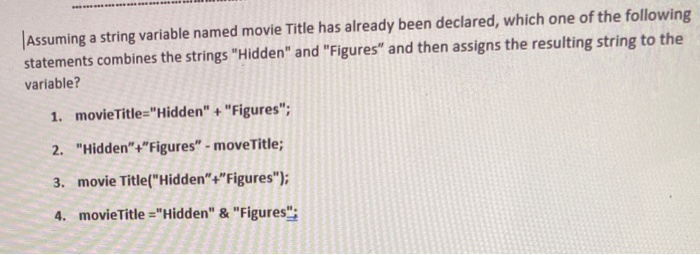In C# (sharp) Assuming a string variable named movie Title has already been declared, which one of the following statements combines the strings "Hidden" and "Figures" and then assigns the resulting string to the variable? 1. movieTitle="Hidden" + "Figures"; 2. "Hidden" +"Figures" - moveTitle; 3. movie Title("Hidden" +"Figures"); 4. movie title="Hidden" & "Figures": Look at the following code: int distance; // Declare distance as an int double half://Declare half as a double distance = 35; // Assign 35 to distance...

• ### The variable cp_arr has been declared as an array of 26 pointers to char

The variable cp_arr has been declared as an array of 26 pointers to char. Allocate 26 character values, initialized to the letters 'A' through 'Z' and assign their pointers to the elements of cp_arr (in that order). int *arr1={'A','B','C','D','E','F','G','H','I','J','K','L','M','N','O','P','Q','R','S','T','U','V','W','X','Y','Z'}; arr1=cp_arr; for(int i = 0; i < 26; i++) cout << cp_arr[i]; is the newest, char a ='A', b = 'B', c = 'C', d='D', e='E', f='F', g='G', h='H', i='I', j='J', k='K',l='L', m='M', n='N', o='O', p='P', q='Q', r='R', s='S', t='T', u='U',...